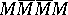# Lewy operator and Mizohata operator

In the mid 1950s, B. Malgrange and L. Ehrenpreis showed that one can solve all linear constant-coefficients partial differential equations on ${\bf R} ^ { n }$ (cf. also Malgrange–Ehrenpreis theorem; Linear partial differential equation). The question of solvability for variable coefficients was posed (avoiding singular points, as needed even in the theory of ordinary differential equations). H. Lewy [a5] found the following example, which at the time (1956) was astonishing. In $3$ variables $( x , y , t )$, let $L$ be the operator (called the Lewy operator):

\begin{equation*} L = \frac { \partial } { \partial x } + i \frac { \partial } { \partial y } - 2 i ( x + i y ) \frac { \partial } { \partial t }. \end{equation*}

The Cauchy–Kovalevskaya theorem applies to this operator. So, if $g$ is a real-analytic function (cf. Class of differentiability) defined (say) near $0$, then the equation $L ( u ) = g$ can be solved near $0$, with $u$ real analytic. But, there exists a $C ^ { \infty }$-function $g$ such that the equation $L ( u ) = g$ cannot be solved in any neighbourhood of (say) $0$, even with $u$ a distribution (cf. also Generalized function).

Later, it was noticed that the same phenomenon occurs, near any point $( 0 , y )$, for the even simpler (embarrassingly simple!) operator, in $2$ variables (called the Mizohata operator):

\begin{equation*} M = \frac { \partial } { \partial x } + i x \frac { \partial } { \partial y }. \end{equation*}

Both operator have clear connections with complex analysis (cf. Functions of a complex variable, theory of).

The Lewy operator is the tangential Cauchy–Riemann operator (cf. Bergman kernel function) on the "Heisenberg group" , the hypersurface in $\mathbf{C} ^ { 2 }$ parametrized by $( x , y , t ) \mapsto ( z , w ) = ( x + i y , t + i | z | ^ { 2 } )$. This simply means that the functions $z$ and $w$ are in the kernel of $L$. So, in other coordinates, $L$ is the Cauchy–Riemann vector field on the unit sphere in $\mathbf{C} ^ { 2 }$ (the tangential operator characterizing holomorphic functions). An earlier paper by Lewy was [a4]!

The Mizohata operator can be obtained (up to a factor) by pulling back the standard Cauchy–Riemann operator on $\mathbf{C}$ via the singular change of variables: $( x , y ) \mapsto ( x ^ { 2 } / 2 + i y )$. Notice that indeed $L ( x ^ { 2 } / 2 + i y ) = 0$.

This connection with complex analysis allows simple, and transparent, proofs of the non-local solvability (in particular, via the obvious non-propagation of singularities for the adjoint operators).

If one decomposes $L$ or $M$ into real part and imaginary part $X + i Y$, the commutator of $X$ and $Y$ does not belong to the linear span of $X$ and $Y$ (for $x = 0$ in case of the Mizohata operator). L.V. Hörmander understood that this fact was crucial and he showed that, more generally, a similar condition on the commutator of the higher-order terms for general linear differential operators implies non-local solvability. See [a3], Chap. 6.

However, real operators may also be "without solutions" , since as pointed out by F. Treves in 1962, the operator $L \overline{L} \overline{L} L$ is a real operator (and so is).

The question of local solvability for vector fields is totally understood thanks to L. Nirenberg and Treves (condition P), [a8], [a11], Chap. VIII. In $\mathbf{R} ^ { 2 }$, the operator $M _ { k } = \partial / \partial x + i x ^ { k } \partial / \partial y$, $k \in \mathbf{N}$, is locally solvable at $0$ if and only if $k$ is even. Again, $M _ { k }$ (see [a6], Appendix) is related to the Cauchy–Riemann operator via the mapping $( x , y ) \mapsto ( x ^ { k + 1 } / ( k + 1 ) + i y )$ which is one-to-one if $k$ is even. The kernel of $M _ { k }$ consists of functions which are holomorphic functions of $( x ^ { k + 1 } / ( k + 1 ) + i y )$, and this can be taken as the beginning of a whole theory, the theory of hypo-analytic structures initiated by M.S. Baouendi and Treves [a1], [a11].

The Lewy operator and the Mizohata operator are much more similar than it may look. Indeed, they are micro-locally equivalent ([a2], Sect. 9, [a10], Chap. IX), and in fact they serve as micro-local model for non-Levi degenerate CR-structures.

Perturbations of the Lewy operator and of the Mizohata operator are of interest. See [a7], Chap. 1. One can construct arbitrary small perturbations of these operators, still as complex vector fields, in $\mathbf{R} ^ { 3 }$ and $\mathbf{R} ^ { 2 }$ respectively, in such a way that the only functions annihilated by these operators will be those which are constant. This gives Nirenberg's example of a strictly pseudo-convex non-embeddable CR-structure (real dimension $3$, CR dimension $1$).

However the Lewy and the Mizohata operators differ strikingly from the point of view of their small perturbations, for the uniqueness in the Cauchy problem (references, and an elementary exposition, can be found in [a9]).

How to Cite This Entry:
Lewy operator and Mizohata operator. Encyclopedia of Mathematics. URL: http://encyclopediaofmath.org/index.php?title=Lewy_operator_and_Mizohata_operator&oldid=51673
This article was adapted from an original article by Jean-Pierre Rosay (originator), which appeared in Encyclopedia of Mathematics - ISBN 1402006098. See original article